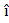# A particle moves from the point (2.0) m, at t = 0, with an initial velocity (5.0+4.0) ms−1. It is acted upon by a constant force which produces a constant acceleration (4.04.0) ms−2. What is the distance of the particle from the origin at time 2 s? a. 20m b. 10m c. 5 m d. 15 m

## Question ID - 50378 :- A particle moves from the point (2.0) m, at t = 0, with an initial velocity (5.0+4.0) ms−1. It is acted upon by a constant force which produces a constant acceleration (4.04.0) ms−2. What is the distance of the particle from the origin at time 2 s? a. 20m b. 10m c. 5 m d. 15 m

3537= (5+ 4)2 +(4+ 4)4

= 10+ 8+ 8+ 8= 18+ 16= 20+ 20= 20Next Question :

A monochromatic light is incident at a certain angle on an equilateral triangular prism and suffers minimum deviation. If the refractive index of the material of the prism is, then the angle of incidence is :

 a. 30° b. 45° c. 90° d. 60°